# NCERT Solutions For Class 6 Maths Chapter 14 Practical Geometry

NCERT Solutions For Class 6 Maths Chapter 14 Practical Geometry

### NCERT Solutions For Class 6 Maths Chapter 14 Practical Geometry Ex 14.1

NCERT Solutions For Class 6 Maths Chapter 14 Practical Geometry Ex 14.1

Exercise 14.1

Ex 14.1 Class 6 Maths Question 1.
Draw a circle of radius 3.2 cm.
Solution:
Step I : Mark a point O as a centre.
Step II: Open the compass up to the given radius 3.2 cm.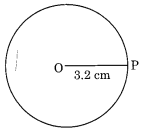Step III : Put the needle of the compass at the centre O.
Step IV : Holding the top of the compass take one full round with pencil. The figure thus obtained is the required circle of radius 3.2 cm.

Ex 14.1 Class 6 Maths Question 2.
With the same centre O, Draw two circles of radius 4 cm and 2.5 cm.
Solution:
Step I : Take centre O and open the compass up to 4 cm.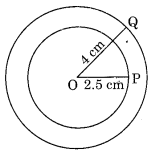Step II : Draw a circle keeping the needle fixed at O.
Step III : Take the same centre O and open the compass up to 2.5 cm, and draw another circle.
The figure shows the required two circles with the same centre.

Ex 14.1 Class 6 Maths Question 3.
Draw a circle and any two its diameters. If you join the ends of these diameters, what is the figure obtained? What figure is obtained if the diameters are perpendicular to each other? How do you check your answer?
Solution: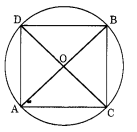(i) Draw a circle with centre 0 with suitable radius.
(ii) AB and CD are any two diameters.
(iii) On joining the end points of the diameters, we get a quadrilateral ACBD.
(iv) We note that OA = OB = OC = OD [Same radius]
and AC = DB, AD = BC
∠A = ∠C = ∠B = ∠D = 90°
Thus ACBD is a rectangle.
Again if the diameters are perpendicular to each other then on measuring, we get
AC = DB = AD = BC
Thus, ACBD is a square.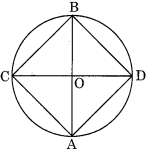Ex 14.1 Class 6 Maths Question 4.
Draw any circle and mark points A, B and C such that
(a) A is on the circle
(b) B is in the interior of the circle
(c) C is in the exterior of the circle.
Solution:
Draw a circle with centre 0 and a suitable radius.
Here
(a) A is on the circle.
(b) B is in the interior of the circle.
(c) C is in the exterior of the circle.Ex 14.1 Class 6 Maths Question 5.
Let A, B be the centres of the two circles of equal radii. Draw them so that each one of them passes through the centre of the other. Let them intersect at C and D.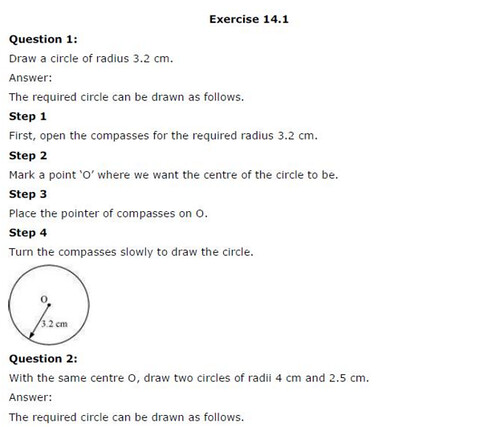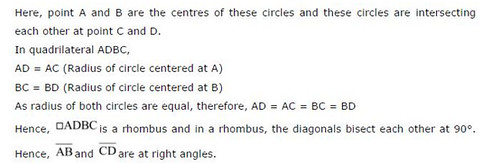## SabDekho

The Complete Educational Website